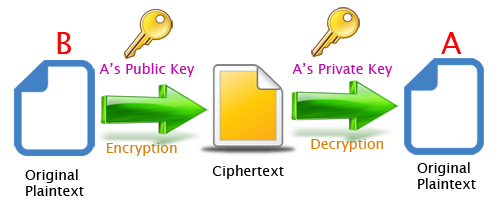# Mengapa RSA tidak cocok untuk enkripsi file binary

By | January 15, 20211,262 Views

## Sinopsis

Mengapa RSA tidak cocok / pas untuk enkripsi sebuag file binary Berikut penjelasannya:

RSA is not designed to encrypt files. Just use a symmetric algorithm (AES, Blowfish, etc.) to encrypt your file, and use RSA only on that symmetric key, if you need symmetric encryption. You cannot encrypt a file using RSA because RSA (well, to be more precise, the implementation of RSA in Java) does not let you encrypt more data than the length of the key. For a 1024 bits key, you can only encrypt 1024 bits that is to say 128 bytes (actually a bit less for padding reasons). In all cases, it is bad practice to encrypt a large piece of data using a public-key algorithm (asymmetric cryptography) for two main reasons.

1. The is no practical, appropriate and secure cryptographic mode/padding to encrypt large amounts of data using RSA (ie it is not really secure to do that).
2. Public-key algorithms require a large key to be secure (1024 bits, 2048 bits) and are therefore much slower than symmetric-key algorithms (which only require 128 to 256 bits keys to be secure).

If you want more details on why you should not use solely RSA to encrypt large amounts of data, see these two great stacktexchange posts :

You cannot encrypt a file using RSA because RSA (well, to be more precise, the implementation of RSA in Java) does not let you encrypt more data than the length of the key. For a 1024 bits key, you can only encrypt 1024 bits that is to say 128 bytes (actually a bit less for padding reasons). In all cases, it is bad practice to encrypt a large piece of data using a public-key algorithm (asymmetric cryptography) for two main reasons. The is no practical, appropriate and secure cryptographic mode/padding to encrypt large amounts of data using RSA (ie it is not really secure to do that).
Public-key algorithms require a large key to be secure (1024 bits, 2048 bits) and are therefore much slower than symmetric-key algorithms (which only require 128 to 256 bits keys to be secure).

If you want more details on why you should not use solely RSA to encrypt large amounts of data, see these two great stacktexchange posts :

If you want to encrypt a large amount of data, the standard way to proceed is to generate a session key (a cryptographically secure random number used once). You encrypt the session key with the public key. Then you encrypt the file (the large amount of data) with a symmetric algorithm (such AES) using the unencrypted session key. You then store the encrypted session key and the encrypted data altogether in the final file. That’s the way PGP (or GnuPG) proceeds when it sends an encrypted mail. SSL/TLS also works in a similar way. Lastly, properly using cryptography is complicated (pretty much anything can create a security flaw : encryption modes, padding, etc…) so I would advise you to be very careful and make sure your code is going to be reviewed by someone knowledgeable in crypto matters. Here is a piece of code that shows the general process :

```// 1. Generate a session key
KeyGenerator keyGen = KeyGenerator.getInstance("AES");
keyGen.init(128)
SecretKey sessionKey = keyGen.generateKey();

// 2. Encrypt the session key with the RSA public key
Cipher rsaCipher = Cipher.getInstance("RSA");
rsaCipher.init(Cipher.ENCRYPT_MODE, rsaPublicKey)
byte[] encryptedSessionKey = rsaCipher.doFinal(sessionKey.getEncoded());

// 3. Encrypt the data using the session key (unencrypted)
aesCipher.init(Cipher.ENCRYPT_MODE, sessionKey); <-- sessionKey is the unencrypted
//                                                   session key.
// ... use aesCipher to encrypt your data

// 4. Save the encrypted data along with the encrypted
// session key (encryptedSessionKey).
// PLEASE NOTE THAT BECAUSE OF THE ENCRYPTION MODE (CBC),
// YOU ALSO NEED TO ALSO SAVE THE IV (INITIALIZATION VECTOR).
// aesCipher.aesCipher.getParameters().